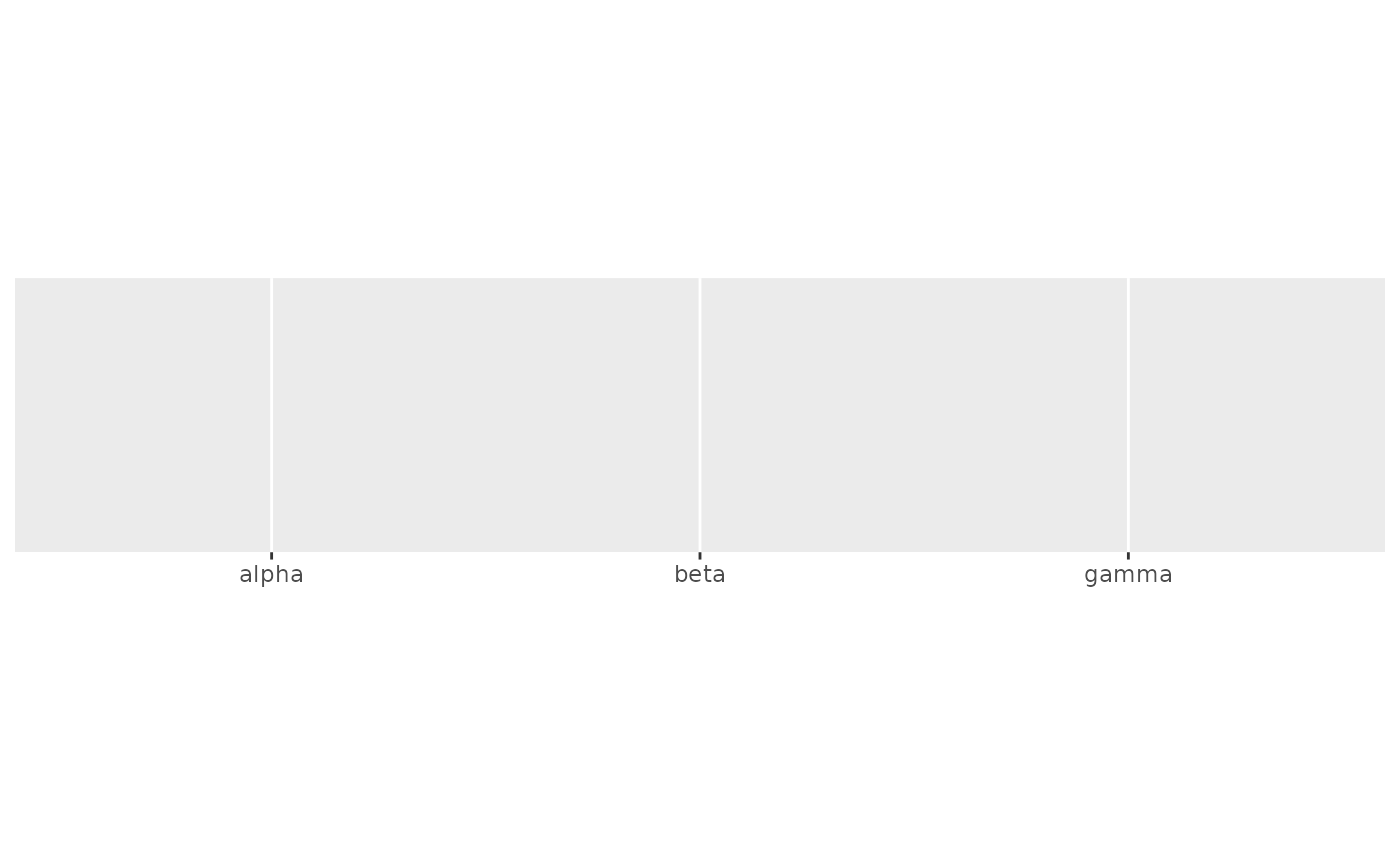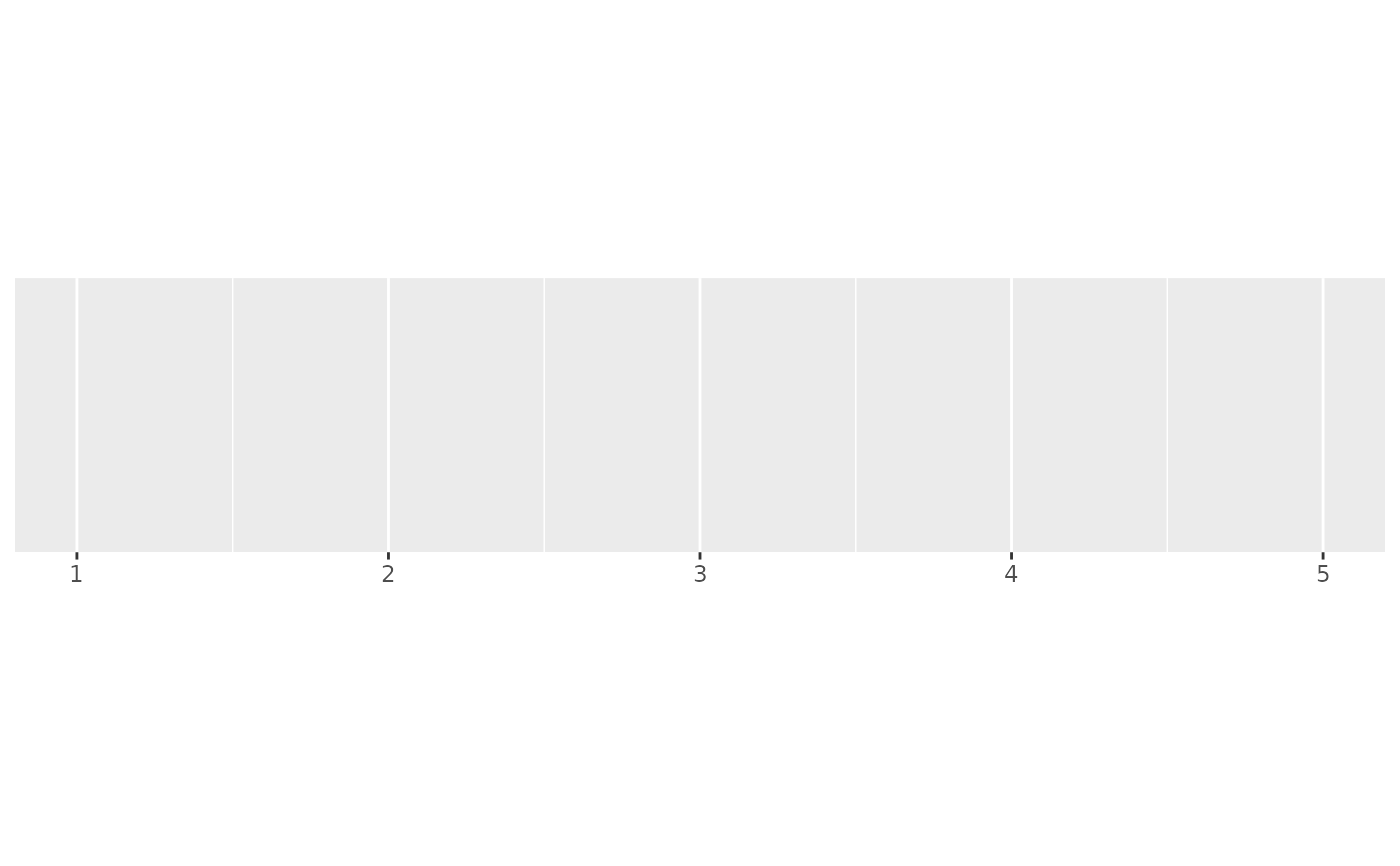label_parse() produces expression from strings by parsing them; label_math() constructs expressions by replacing the pronoun .x with each string.

label_parse()

label_math(expr = 10^.x, format = force)

parse_format()

math_format(expr = 10^.x, format = force)

## Arguments

expr expression to use another format function to apply prior to mathematical transformation - this makes it easier to use floating point numbers in mathematical expressions.

## Value

All label_() functions return a "labelling" function, i.e. a function that takes a vector x and returns a character vector of length(x) giving a label for each input value.

Labelling functions are designed to be used with the labels argument of ggplot2 scales. The examples demonstrate their use with x scales, but they work similarly for all scales, including those that generate legends rather than axes.

## Old interface

parse_format() and math_format() was retired; please use label_parse() and label_math() instead.

## See also

plotmath for the details of mathematical formatting in R.

Other labels for continuous scales: label_bytes(), label_dollar(), label_number_auto(), label_number_si(), label_ordinal(), label_percent(), label_pvalue(), label_scientific()

Other labels for discrete scales: label_wrap()

## Examples

# Use label_parse() with discrete scales
greek <- c("alpha", "beta", "gamma")
demo_discrete(greek)
#> scale_x_discrete()demo_discrete(greek, labels = label_parse())
#> scale_x_discrete(labels = label_parse())# Use label_math() with continuous scales
demo_continuous(c(1, 5))
#> scale_x_continuous()demo_continuous(c(1, 5), labels = label_math(alpha[.x]))
#> scale_x_continuous(labels = label_math(alpha[.x]))